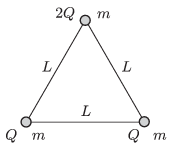Mathematical and Physical Journal
for High Schools
Issued by the MATFUND Foundation
 Already signed up? New to KöMaL?

#Problem P. 4734. (April 2015)

P. 4734. Three small positively charged balls of mass $\displaystyle m$ are attached by massless insulating threads of length $\displaystyle L$ and are placed to a horizontal smooth surface made of some insulating material. Two of the balls both have a charge of $\displaystyle Q$ and the third is charged to $\displaystyle 2Q$. At an instant the thread between the two balls of charge $\displaystyle Q$ is cut off.$\displaystyle a)$ What are the velocities of the balls at the instant when the ball of charge $\displaystyle 2Q$ is the fastest?

$\displaystyle b)$ What is the tension in the threads at this moment?

$\displaystyle c)$ What are the displacements of the balls at this moment?

(5 pont)

Deadline expired on May 11, 2015.

### Statistics:

 41 students sent a solution. 5 points: Asztalos Bogdán, Balogh Menyhért, Bartók Imre, Bekes Nándor, Bencsik Bálint, Csathó Botond, Csire Roland, Csorba Benjámin, Fehér Balázs, Fekete Panna, Forrai Botond, Fülöp Erik, Holczer András, Jakus Balázs István, Kaposvári Péter, Kasza Bence, Kormányos Hanna Rebeka, Marosvári Kristóf, Németh Flóra Boróka, Öreg Botond, Pázmán Előd, Sal Kristóf, Szántó Benedek, Szentivánszki Soma , Szépfalvi Bálint. 4 points: Berta Dénes, Blum Balázs, Büki Máté, Kovács Péter Tamás, Körtefái Dóra, Nagy 555 Botond, Olosz Balázs, Páhoki Tamás, Radnai Bálint, Tanner Martin, Varga-Umbrich Eszter. 3 points: 5 students.

Problems in Physics of KöMaL, April 2015